# Expression of a variable from the formula + natural numbers - math problems

#### Number of problems found: 32

• Sum of four numbers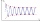The sum of four consecutive natural numbers is 114. Find them.
• The sumThe sum of the squares of two immediately following natural numbers is 1201. Find these numbers.
• Sum of the seventeen numbersThe sum of the 17 different natural numbers is 154. Determine the sum of the two largest ones.
• Negative difference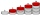Find four arithmetic progression members between 7 and -6.
• Even / odd numbers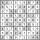a / Using variable n write two consecutive odd numbers. b / the sum of three consecutive odd numbers is 333. What are this numbers?
• What areWhat are the four terms between 4 and 19?
• DigitsShow that if x, y, z are 3 consecutive nonzero digits, zyx-xyz = 198, where zyx and xyz are three-digit numbers created from x, y, z.
• Simple sequence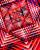Continue with this series of numbers: 1792,448, 112, _, _
• RectanglesThe perimeter of a rectangle is 90 m. Divide it into three rectangles, the shorter side has all three rectangles the same, their longer sides are three consecutive natural numbers. What is the dimensions of each rectangle?
• AP membersWhat is the value of x2, x3, x4, x5…of the terms (of arithmetic progression) when x1 = 8 and x6 = 20?
• Unknown number 716% of the unknown number is by 21 less than unknown number itself. Determine the natural unknown number.
• 1 page1 page is torn from the book. The sum of the page numbers of all the remaining pages is 15,000. What numbers did the pages have on the page that was torn from the book?
• Two-digit numberDigit sum of thinking two-digit natural number is 11. When it exchanging a sequence of digits, given a number which is 27 less than the thinking number. Find out which number I think.
• AlleyAlley measured a meters. At the beginning and end are planted poplar. How many we must plant poplars to get the distance between the poplars 15 meters?
• QuotientFind quotient before the bracket - the largest divisor 51 a + 34 b + 68 121y-99z-33
• Four integersFnd four consecutive integers so that the product of the first two is 70 times smaller than the product of the next two.
• LibraryNew books were purchased for the library. Five-eighths were professional books, one-fifth were encyclopedias, and 231 books were dictionaries. How many professional books were there?
• Twenty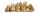Twenty rabbits are put in 4 cells so that there are a different number of rabbits in each cell contains at least 3 rabbits. What is the largest possible number of rabbits in one cell?
• Rectangle diagonalsIt is given a rectangle with an area of 24 cm2 a circumference of 20 cm. The length of one side is 2 cm larger than the length of the second side. Calculate the length of the diagonal. Length and width are yet expressed in natural numbers.
• Triple and quadruple rooms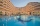Up to 48 rooms, some of which are triple and some quadruple, accommodated 173 people so that all beds are occupied. How many triple and how many quadruple rooms were there?

Do you have an interesting mathematical word problem that you can't solve it? Submit a math problem, and we can try to solve it.

We will send a solution to your e-mail address. Solved examples are also published here. Please enter the e-mail correctly and check whether you don't have a full mailbox.

Please do not submit problems from current active competitions such as Mathematical Olympiad, correspondence seminars etc...
Expression of a variable from the formula - math problems. Natural numbers - math problems.